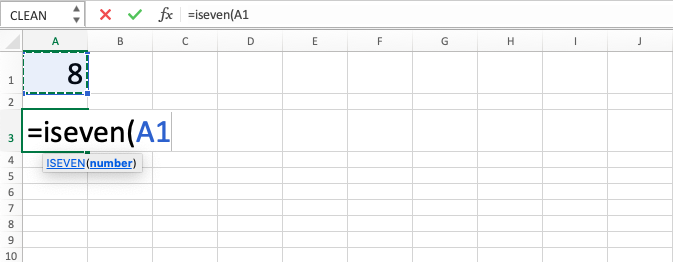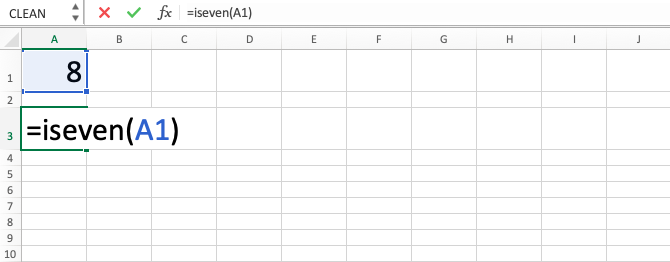ISEVEN Excel Formula - Compute Expert

# ISEVEN Excel Formula

In this tutorial, you will learn the use of the ISEVEN excel formula. ISEVEN function in excel is useful to evaluate numeric data whether it is an even digit and give TRUE or FALSE result depending on the evaluation result.

## Why do We Need to Learn ISEVEN Function in Excel?

In numeric data processing on a spreadsheet, sometimes we need to see whether the numbers that we have are even or not. This can be needed for various purposes. Of course, it will be hard to do it manually if there are hundreds or thousands of numbers to identify whether each of them is even or not. The process can be long and become inaccurate if we do it manually.

As an example of the identification need, imagine we are making product division to be delivered to two company regions. The division to one region is marked with the even number on the product code thus we need to identify the code from all of the products so we can decide correctly for their delivery destination. If we want to mark even product codes in the products to add the region delivery notes, then we must see the codes one by one. If it cannot be done automatically, then of course it will be much harder especially if there are hundreds or thousands of products that must be identified to add the notes.

To help automate the identification process, then you can use ISEVEN excel formula. ISEVEN function in excel will help to evaluate numeric data on the spreadsheet and give TRUE if it is even. It will be useful to be learned and understood if you often deal with numeric data on the spreadsheet especially in the even and odd groupings of it.

## What is ISEVEN Excel Formula?

ISEVEN excel formula is a formula with the usage objective to evaluate numeric data and give TRUE if it is even and FALSE if not. Just as other IS functions in the spreadsheet, this function is often combined with IF formula in its usage.

Generally, its input can be explained as follows:

=ISEVEN(value)

Note:
value = a numeric data that we want to see the value of, whether it is even or not

## How to Use ISEVEN Function in Excel?

The following will explain how to write the ISEVEN function in excel from the start. This formula only needs one input which is the numeric data for it to evaluate (usually the input here is a cell coordinate or a formula that can produce the data).

## Using ISEVEN Excel Formula

1. Type equal sign ( = ) in the cell where you want to put the result in2. Type ISEVEN (can be with large and small letters) and open bracket sign after =3. Input the number / the process that produces the number / cell coordinate where it is located after open bracket sign4. Input close bracket sign5. Press Enter
6. The process is done!## Exercise

Now that you have done the learning of how to use the ISEVEN function in excel, it is time for you to deepen your understanding by doing the following exercise!

### Questions

Answer each question in the gray-colored cells on the appropriate row number using the ISEVEN function in excel!
1. Is the cell content on the left even? Answer with TRUE or FALSE
2. Is the cell content on the left even? Answer with “V” if yes or “X” if not. Use the IF function too to help you!
3. Add the cell content on the left with 1000 if it is even and make it to 0 if it is FALSE! Use IF again to answer this question!

• If you give non-numeric data input in ISEVEN excel formula, then you can get a #VALUE error
• If you want to evaluate whether a data is odd or not, then you can use the ISODD formula

Get updated excel info from Compute Expert by registering your email. It's free!